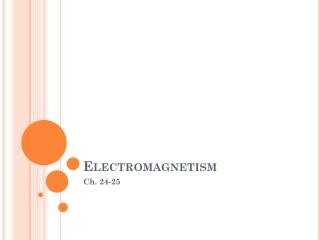DownloadDownload PresentationElectromagnetism

# Electromagnetism

Download Presentation## Electromagnetism

- - - - - - - - - - - - - - - - - - - - - - - - - - - E N D - - - - - - - - - - - - - - - - - - - - - - - - - - -
##### Presentation Transcript

1. Electromagnetism Ch. 24-25

2. Electromagnetism • Electromagnetism describes the relationship between electricity and magnetism. • We use electromagnets to generate electricity, store memory on our computers, generate pictures on a television screen, diagnose illnesses, and in just about every other aspect of our lives that depends on electricity. • Electromagnetism works on the principle that an electric current through a wire generates a magnetic field. • Maglev Train

3. Electromagnets • This magnetic field is the same force that makes metal objects stick to permanent magnets. • In a bar magnet, the magnetic field runs from the north to the south pole. • In a wire, the magnetic field forms around the wire. If we wrap that wire around a metal object, we can often magnetize that object. In this way, we can create an electromagnet.

4. Electromagnets When DC electricity is passed through a wire, a magnetic field rotates around the wire in a specific direction. “Right Hand Rule”-If you take your right hand and wrap it around the wire, with your thumb pointing in the direction of the electrical current (positive to negative), then your fingers are pointing in the direction of the magnetic field around the wire.

5. Electromagnetic Induction • When a wire moves in a magnetic field, a force acts on the charges in the wire. • Work is done on the charges. • The Fun Side of Magnets

6. Electric power • Current x voltage • Unit • Watts • Kilowatt-hour • Energy delivered in 1 hour at the rate of 1 kW

7. Difference in AC/DC Current • Electricity flows in two ways; either in alternating current (AC) or in direct current (DC). • Therefore, the difference between AC and DC has to do with the direction in which the electrons flow. • In DC, the electrons flow steadily in a single direction, or "forward." • In AC, electrons keep switching directions, sometimes going "forward" and then going "backward.” • In the US, electric utilities use a 60-Hz frequency, meaning that the current alternates direction (forward to backward and back to forward) 60 times in one second.

8. Today we will discuss Transformers… Which one though? The Left or the right picture?

9. Transformers • A transformer is a device for changing electrical energy. It transfers electrical energy from one alternating circuit to another with a change in voltage, current, phase, or impedance.

10. Transformers The primary voltage (on the left) induces a magnetic field in the core, which creates the secondary voltage (on the right). What makes transformers so useful is that if you change the number of turns from one side to the other, you change the voltage in the wire on the right! Transformers can change a high voltage to a lower one, or a low voltage to a higher one.

11. Step Up • A step-up transformer converts a low voltage to a higher one. • If you increase the number of turns on the right, the voltage coming off the transformer will increase in proportion. • The right side has 4 times more turns so the voltage on the right has increased 4 times. • So the voltage has been stepped up by a factor of 4.

12. Step Down Transformer • Step-down transformer reduces voltage. • If you decrease the number of turns on the right, the voltage coming off the transformer will decrease in proportion. • The right side has 1/5 the number of turns, so the voltage is only 1/5 as large. • So the voltage has been stepped down by 5.

13. Transformers Math • We can express the relationship between the primary and the secondary coils like this Is = Vs = Ns IpVpNp secondary current = secondary voltage = number of turns on secondary coil primary current primary voltage number of turns on primary coil Also keep in mind that Pp = Ps ( power of primary equals the primary of the secondary) * VpIp = VsIs

14. Why Transmit with AC vs. DC • Advantages to using AC: Transformers can only work using AC b/c the magnetic field has to be constantly changing in order to induce the secondary current. Due to the constant change of direction of the electrons in AC, the magnetic field is always changing as well. • By using a step-up transformer to hike the voltage, we are able to lower the current and by lowering current, we are able to use thinner wires. • Ultimately, we are able to transfer power farther (higher voltage travels farther), for less money (cost of wire), with better efficiency (less power lost to heat caused by resistance).

15. DC Motors

16. What is the function of the Split-ring Commutator? • If you look closely, you will see that it switches the flow of current half-way through the rotation of the armature. • By doing so, it reverses the magnetic field produced around the armature. This reversal at the exact right moment makes the armature continue to be pushed/pulled by the forces of the magnets.